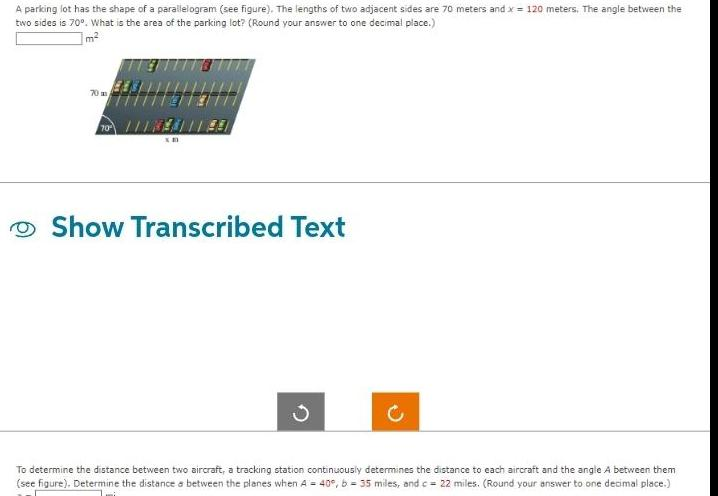Question:

# A parking lot has the shape of a parallelogram see figure

Last updated: 8/15/2023A parking lot has the shape of a parallelogram see figure The lengths of two adjacent sides are 70 meters and x 120 meters The angle between the two sides is 70 What is the area of the parking lot Round your answer to one decimal place m 70 4411 40 Show Transcribed Text C To determine the distance between two aircraft a tracking station continuously determines the distance to each aircraft and the angle A between them see figure Determine the distance between the planes when A 40 b 35 miles and c 22 miles Round your answer to one decimal place